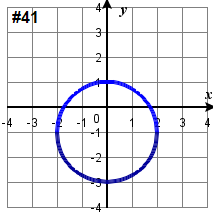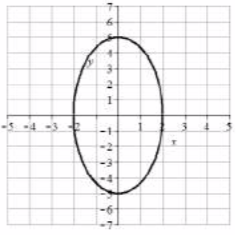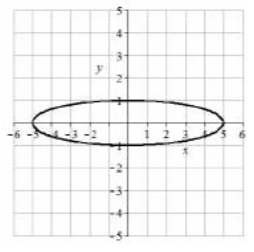# 3.2e: Circle Exercises.

$$\newcommand{\vecs}{\overset { \rightharpoonup} {\mathbf{#1}} }$$ $$\newcommand{\vecd}{\overset{-\!-\!\rightharpoonup}{\vphantom{a}\smash {#1}}}$$$$\newcommand{\id}{\mathrm{id}}$$ $$\newcommand{\Span}{\mathrm{span}}$$ $$\newcommand{\kernel}{\mathrm{null}\,}$$ $$\newcommand{\range}{\mathrm{range}\,}$$ $$\newcommand{\RealPart}{\mathrm{Re}}$$ $$\newcommand{\ImaginaryPart}{\mathrm{Im}}$$ $$\newcommand{\Argument}{\mathrm{Arg}}$$ $$\newcommand{\norm}{\| #1 \|}$$ $$\newcommand{\inner}{\langle #1, #2 \rangle}$$ $$\newcommand{\Span}{\mathrm{span}}$$ $$\newcommand{\id}{\mathrm{id}}$$ $$\newcommand{\Span}{\mathrm{span}}$$ $$\newcommand{\kernel}{\mathrm{null}\,}$$ $$\newcommand{\range}{\mathrm{range}\,}$$ $$\newcommand{\RealPart}{\mathrm{Re}}$$ $$\newcommand{\ImaginaryPart}{\mathrm{Im}}$$ $$\newcommand{\Argument}{\mathrm{Arg}}$$ $$\newcommand{\norm}{\| #1 \|}$$ $$\newcommand{\inner}{\langle #1, #2 \rangle}$$ $$\newcommand{\Span}{\mathrm{span}}$$$$\newcommand{\AA}{\unicode[.8,0]{x212B}}$$

### A: Write the Equation for a Circle from a Description

Exercise $$\PageIndex{A}$$

1. Write an equation of the circle centered at (8 , -10) with radius 8.

2. Write an equation of the circle centered at (-9, 9) with radius 16.

1. $$(x-8)^2+(y+10)^2 = 64$$

### B: Construct an equation for a circle from a graph

Exercise $$\PageIndex{B}$$

$$\bigstar$$ State the center and radius of the circle  graphed below and construct the equation for it.

 3.4.5.6.3. Center $$(2, 1)$$, radius  $$r = 2$$, $$(x - 2)^{2} + (y + 1)^{2} = 4$$ 5. Center $$(-1, 3)$$, radius  $$r = 5$$, $$(x+1)^{2} + (y -3)^{2} = 25$$

### C: Graph a circle given an equation

Exercise $$\PageIndex{C}$$

$$\bigstar$$ State the center and radius of the circle described by the equation and graph it.

 $$\left(x-2\right)^{2} + \left(y+3\right)^{2} = 9$$ $$\left(x+1\right)^{2} + \left(y-2\right)^{2} = 16$$ $$(x - 2)^{2} + (y + 5)^{2} = 4$$  $$(x + 1)^{2} + (y + 5)^{2} = 100$$ $$(x + 9)^{2} + y^{2} = 25$$ $$(x-4)^2+(y+2)^2 = 9$$  $$(x+4)^2 + (y-5)^2 = 42$$ $$(x-3)^2+(y-6)^2 = 20$$   $$\left(x + \frac{5}{2}\right)^2 + \left(y - \frac{1}{2}\right)^2 = \frac{30}{4}$$   $$(x-1)^2 + (y-5)^2 = 5$$   $$\left(x + \frac{1}{2}\right)^{2} + \left(y - \frac{3}{5}\right)^{2} = \frac{161}{100}$$    $$(x - 3)^{2} + (y - 5)^{2} = 65$$   $$x^{2} + (y - 3)^{2} = 0$$   $$x^{2} + (y - 72)^{2} = 4096$$
 11. Center $$(2, -3)$$, radius  $$r = 3$$ 13. Center $$(2, -5)$$, radius  $$r = 2$$ 15. Center $$(-9, 0)$$, radius  $$r = 5$$ 17. Center $$(-4,5)$$, radius  $$r = \sqrt{42}$$ 19. Center  $$\left( -\frac{5}{2}, \frac{1}{2}\right)$$, radius  $$r = \frac{\sqrt{30}}{2}$$ 21. Center  $$\left(-\frac{1}{2}, \frac{3}{5}\right)$$, radius  $$r = \frac{\sqrt{161}}{10}$$ 23. This is not a circle.### D: Graph a circle given an equation in non-standard form

Exercise $$\PageIndex{D}$$

$$\bigstar$$ Complete the square and write the equation in standard form. Then give the center and radius of each circle and graph it.

 $$x^2 + y^2 + 2x + 6y - 6 =0$$ $$x^2 + y^2 + 4x + 2y - 4 =0$$ $$x^2 + y^2 - 6x + 18y - 10 =0$$ $$x^2 + y^2 - 8x + 4y - 5 =0$$ $$x^2 + y^2 + 10x - 12y = 13$$ $$x^2 + y^2 + 12x - 8y = 5$$ $$x^2 + y^2 - 14x - 20y = 20$$ $$x^2 + y^2 - 16x - 10y = 11$$ $$x^2 + y^2 + x + 14y + \dfrac{53}{4} =0$$ $$x^2 + y^2 +16 x + 5y = \tfrac{25}{4}$$ $$x^2 + y^2 + 2y = 3$$ $$x^2 + y^2 + 7x -\tfrac{7}{4} =0$$
 31. $$(x +1)^{2} + (y + 3)^{2} = 16$$,  Center: $$(-1, -3)$$, Radius: $$4$$ 33. $$(x - 3)^{2} + (y + 9)^{2} = 100$$,  Center: $$(-3, 9)$$, R: $$10$$   35. $$(x+5)^{2} + (y -6)^{2} = 74$$,  Center: $$(-5, 6)$$, R: $$\sqrt{74}$$  37. $$(x - 7)^{2} + (y -10)^{2} = 169$$,  Center: $$(7, 10)$$, Radius: $$13$$ 39. $$(x + 0.5)^{2} + (y + 7)^{2} = 36$$,  Center: $$(-\tfrac{1}{2}, -7)$$, Radius: $$6$$  41. $$x^{2} + (y + 1)^{2} = 4$$,  Center: $$(0, -1)$$, Radius = $$2$$### E: Match equations with graphs of ellipses

Exercise $$\PageIndex{E}$$

$$\bigstar$$ Match each graph with one of the equations A–D.

 A. $$\dfrac{x^2}{4} + \dfrac{y^2}{9} = 1$$ B. $$\dfrac{x^2}{9} + \dfrac{y^2}{4} = 1$$ C. $$\dfrac{x^2}{9} + {y^2} = 1$$ D. $${x^2} + \dfrac{y^2}{9} = 1$$ 41.42.43.44.$$\bigstar$$ Match each graph to equations A-H.

 A. $$\dfrac{\left( {x - 2} \right)^2}{4} + \dfrac{{(y - 1)}^2}{9} = 1$$ B. $$\dfrac{\left( {x - 2} \right)^2}{4} + \dfrac{{(y - 1)}^2}{16} = 1$$ C. $$\dfrac{\left( {x - 2} \right)^2}{16} + \dfrac{{(y - 1)}^2}{4} = 1$$ D. $$\dfrac{\left( {x - 2} \right)^2}{9} + \dfrac{{(y - 1)}^2}{4} = 1$$ E. $$\dfrac{\left( {x + 2} \right)^2}{4} + \dfrac{{(y + 1)}^2}{9} = 1$$ F. $$\dfrac{\left( {x + 2} \right)^2}{4} + \dfrac{{(y + 1)}^2}{16} = 1$$ G. $$\dfrac{\left( {x + 2} \right)^2}{16} + \dfrac{{(y + 1)}^2}{4} = 1$$ H. $$\dfrac{\left( {x + 2} \right)^2}{9} + \dfrac{{(y + 1)}^2}{4} = 1$$ 45.46.47.49.49.50.51.52.41. D      43. B      45. B      47. C      49. F      51. G

### F: Write the equation for an ellipse given a graph

Exercise $$\PageIndex{F}$$

$$\bigstar$$ Write an equation for the graph.

 53.54.55.56.$$\bigstar$$ Find the standard form of the equation for an ellipse satisfying the given conditions.

 57. Center (0,0), horizontal radius = $$32$$, vertical radius = $$7$$ 58. Center (0,0), horizontal radius = $$9$$, vertical radius = $$18$$ 59. Center (0,0), horizontal radius = $$2$$, vertical radius = $$3$$ 60. Center (0,0), horizontal radius = $$4$$, vertical radius = $$4$$ 61. Center (-4, 3), horizontal radius = $$4$$, vertical radius = $$5$$ 62. Center (1, -2), horizontal radius = $$7$$, vertical radius = $$8$$
 53. $$\dfrac{x^2}{16} + \dfrac{y^2}{4} = 1$$ 55. $$(x - 3)^2 + \dfrac{(y + 1)^2}{16} = 1$$ 57. $$\dfrac{x^2}{1024} + \dfrac{y^2}{49} = 1$$ 59. $$\dfrac{x^2}{4} + \dfrac{y^2}{9} = 1$$ 61. $$\dfrac{(x + 4)^2}{16} + \dfrac{(y - 3)^2}{25} = 1$$

### G: Graph an ellipse given an equation

Exercise $$\PageIndex{G}$$: Graph an Ellipse from an Equation in Standard Form

$$\bigstar$$ Find the center, and horizontal and vertical radii. Sketch the graph.

 63. $$\dfrac{x^2}{4} + \dfrac{y^2}{25} = 1$$ 64. $$\dfrac{x^2}{16} + \dfrac{y^2}{4} = 1$$ 65. $$\dfrac{x^2}{4} + y^2 = 1$$ 66. $$x^2 + \dfrac{y^2}{25} = 1$$ 67. $$x^2+ 25y^2 = 25$$ 68. $$16x^2 + y^2 = 16$$ 69. $$16x^2 + 9y^2 = 144$$ 70. $$16x^2 + 25y^2 = 400$$ 71. $$9x^2 + y^2 = 18$$ 72. $$x^2 + 4y^2 = 12$$
 63. Center $$(0, 0)$$, Horiz. radius $$a = 2$$, Vert. radius $$b = 5$$65. Center $$(0, 0)$$, Horiz. radius $$a = 2$$, Vert. radius $$b = 1$$67. Center $$(0, 0)$$, Horiz. radius $$a = 5$$, Vert. radius $$b = 1$$69. Center $$(0, 0)$$, Horiz. radius $$a = 3$$, Vert. radius $$b = 4$$71. Center $$(0, 0)$$, Horiz. radius $$a = \sqrt{2}$$, Vert. radius $$b =3\sqrt{2}$$### H: Graph an ellipse given an equation in non-standard form

Exercise $$\PageIndex{H}$$

$$\bigstar$$ Find the center, and horizontal and vertical radii. Sketch the graph.

 73. $$\dfrac{(x - 1)^2}{25} + \dfrac{(y + 2)^2}{4} = 1$$   74. $$\dfrac{(x + 5)^2}{16} + \dfrac{(y - 3)^2}{36} = 1$$ 75. $$(x + 2)^2 + \dfrac{(y - 3)^2}{25} = 1$$   76. $$\dfrac{(x - 1)^2}{25} + (y - 6)^2 = 1$$ 77. $$4x^2 + 8x + 4 + y^2 = 16$$ 78. $$x^2 + 4y^2 + 16y + 16 = 36$$ 79. $$x^2 + 2x + 4y^2 + 16y = - 1$$ 80. $$4x^2 + 16x + y^2 - 8y = 4$$ 81. $$9x^2 - 36x + 4y^2 + 8y = 104$$ 82. $$4x^2 + 8x + 9y^2 + 36y = - 4$$
 73. Center (1, -2), Horiz. radius $$a = 5$$, Vert. radius $$b = 2$$75. Center (-2, 3), Horiz. radius $$a = 1$$, Vert. radius $$b = 5$$77. Center (-1, 0), Horiz. radius $$a = 2$$, Vert. radius $$b = 4$$79. Center (-1, -2), Horiz. radius $$a = 4$$, Vert. radius $$b = 2$$81. Center (2, -1), Horiz. radius $$a = 4$$, Vert. radius $$b = 6$$